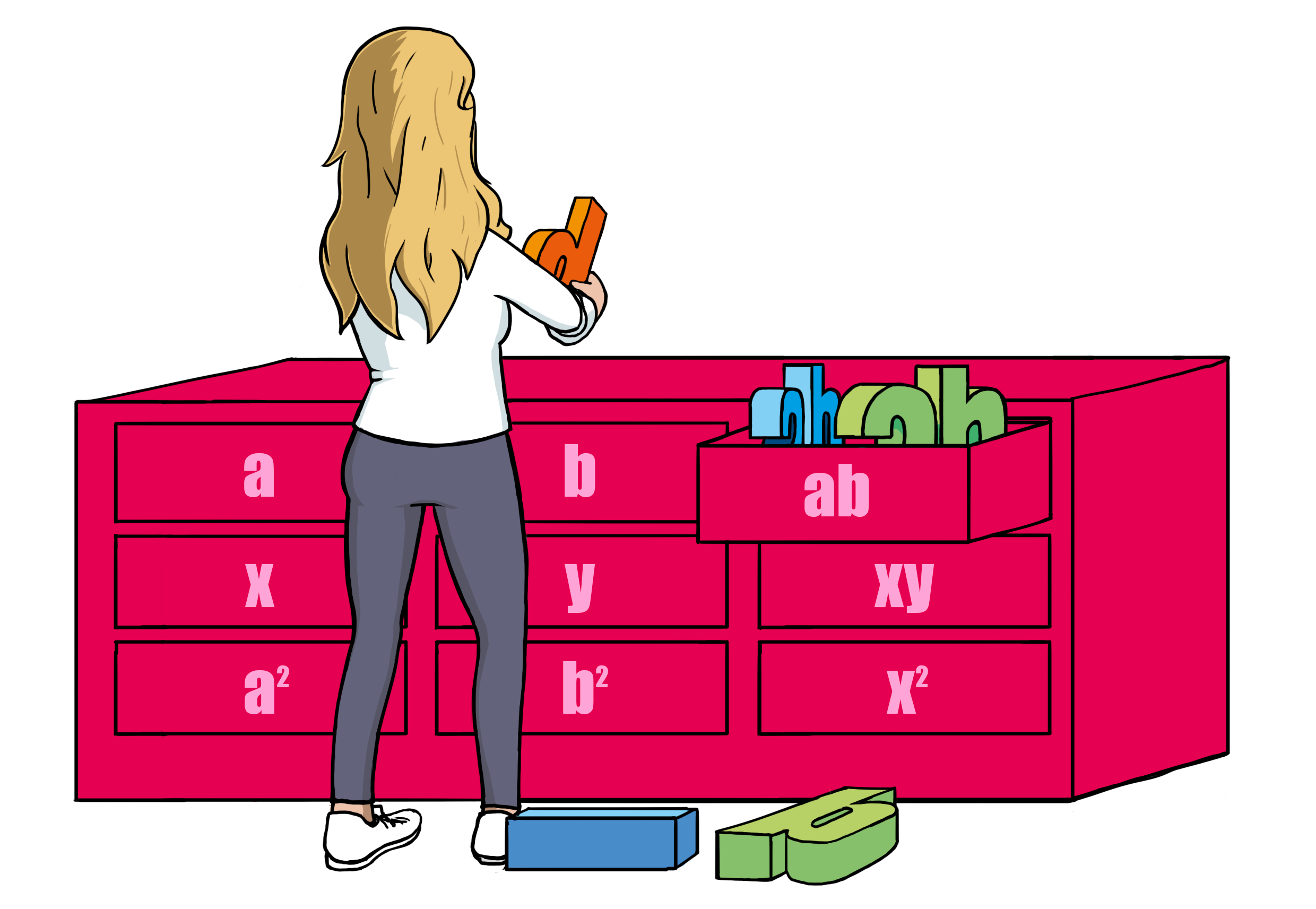# Examples of Multiplying Numbers with Variables

Now you’ll learn about multiplying both positive and negative numbers by both positive and negative expressions with variables in them.

To be comfortable with this you need to be able to handle signs, numbers and variables. For that reason, there is a really nice memory aid that will always give you the correct answer!

Rule

### Sign,Number,Variable

1.
Determine the sign (SIGN).
2.
Multiply the numbers by each other (NUMBER).
3.
Attach the variables (VARIABLE).

Example 1

Multiplying numbers and variables: $\begin{array}{llll}\hfill -2\cdot 2x& =-+2\cdot 2x\phantom{\rule{2em}{0ex}}& \hfill & \phantom{\rule{2em}{0ex}}\\ \hfill & =-4x\phantom{\rule{2em}{0ex}}& \hfill & \phantom{\rule{2em}{0ex}}\end{array}$

Example 2

Multiplying numbers and variables: $\begin{array}{llll}\hfill 1\cdot 7x& =++1\cdot 7x\phantom{\rule{2em}{0ex}}& \hfill & \phantom{\rule{2em}{0ex}}\\ \hfill & =+7x\phantom{\rule{2em}{0ex}}& \hfill & \phantom{\rule{2em}{0ex}}\\ \hfill & =7x\phantom{\rule{2em}{0ex}}& \hfill & \phantom{\rule{2em}{0ex}}\end{array}$

Example 3

Multiplying numbers and variables: $\begin{array}{llll}\hfill 3\cdot \left(-6x\right)& =+-3\cdot 6x\phantom{\rule{2em}{0ex}}& \hfill & \phantom{\rule{2em}{0ex}}\\ \hfill & =-18x\phantom{\rule{2em}{0ex}}& \hfill & \phantom{\rule{2em}{0ex}}\end{array}$

Example 4

Multiplying numbers and variables: $\begin{array}{llll}\hfill -4\cdot \left(-5x\right)& =--4\cdot 5x\phantom{\rule{2em}{0ex}}& \hfill & \phantom{\rule{2em}{0ex}}\\ \hfill & =+20x\phantom{\rule{2em}{0ex}}& \hfill & \phantom{\rule{2em}{0ex}}\\ \hfill & =20x\phantom{\rule{2em}{0ex}}& \hfill & \phantom{\rule{2em}{0ex}}\end{array}$

The first thing you need to decide is whether the answer is positive or negative. To do this you need to look at the signs of all the factors. Then you multiply the factors that are numbers together, so that you end up with the right number of variables. Finally, you add the variable or variables that were part of the multiplication expression.

By following these instructions, you’ll always find the correct answer. Take some time to practice this by doing lots of problems like the ones in the examples above.